# Quantization Error and Signal - to - Noise Ratio calculations

The signal to noise ratio of a quantized signal is 2+6*(no of bits), as shown in the following table.

#### Resolution and Signal to Noise Ratio for signals coded as n bits

bits, n

levels, 2n Weighting of LSB, 2-n SNR, dB
1 2 0.5 8
2 4 0.25 14
3 8 0.125 20
4 16 0.0625 26
5 32 0.03125 32
6 64 0.01563 38
7 128 0.00781 44
8 256 0.00391 50
9 512 0.00195 56
10 1024 0.00098 62
11 2048 0.00048 68
12 4096 0.00024 74
13 8192 0.00012 80
14 16384 0.00006 86
15 32768 0.00003 92
16 65536 0.00001 98

These values are for a signal matched to the full-scale range of the converter.  If a signal with a range of 5V is measured by an 8 bit ADC with a range of 10V then only 7 bits are effectively in use, and a signal to noise ratio of 44 rather than 50 will apply.

#### Proof:

Suppose that the instantaneous value of the input voltage is measured by an ADC with a Full Scale Range of Vfs volts, and a resolution of n bits. The real value can change through a range of q = Vfs / 2n volts without a change in measured value occurring.

The value of the measured signal is Vm = Vs - e, where
Vm is the measured value,
Vs is the actual value, and
e is the error.

The maximum value of error in the measured signal is

emax = (1/2)(Vfs / 2n) or emax = q/2 since q = Vfs / 2n

The RMS value of quantization error voltage is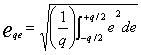whence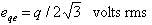The Signal to Noise Ratio (SNR) is defined asIt is normally quoted on a logarithmic scale, in deciBels ( dB ).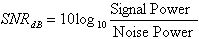or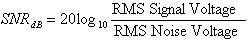The RMS signal voltage is then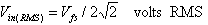The error, or quantization noise signal is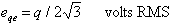Thus the signal - to - noise ratio in dB. is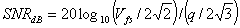since Vfs = 2n q, then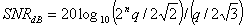which simplifies toN.B. This equation is true only if the input signal is exactly matched to the Full Scale Range of the converter. For signals whose amplitude is less than the FSR the Signal - to - Noise Ratio will be reduced.

Download a .pdf file of the analysis of quantization error and signal to noise ratio